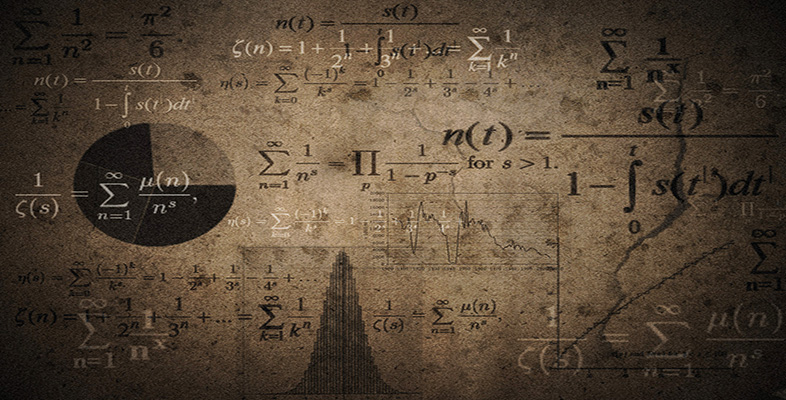Language, notation and formulas

Start this free course now. Just create an account and sign in. Enrol and complete the course for a free statement of participation or digital badge if available.

Free course

# 3 Formulas

## 3.1 Word formulas

A formula is a rule or a generalisation. Word formulas – formulas that use English words rather than mathematical symbols – are so much a part of life that people often use them without realising that they are doing so. Here are some examples.

• The cost of a purchase of oranges is the price per orange times the number of oranges.

• The total cost of petrol is the price of petrol per litre times the number of litres.

• The average speed is the distance travelled divided by the time taken.

• The number of tins of paint needed to cover a wall is the area of the wall divided by the area covered by one tin of paint.

These word formulas can be written more clearly using mathematical symbols. Notice how brackets can be used for clarity.

• Cost of oranges bought = (price per orange) × (number of oranges).

• Cost of petrol = (price of petrol per litre) × (number of litres).

• Average speed = (distance travelled)/(time taken).

• Number of tins of paint needed = (area of wall)/(area covered by one tin).

Sometimes letters are used as shorthand in formulas.

### Example 7

At constant speed, ‘distance is the product of speed and time’. Write this as a word formula and then shorten it by using symbols: d for distance, s for speed and t for time.

distance = (speed) × (time)

d = s × t or d = st

MU120_4M6